Question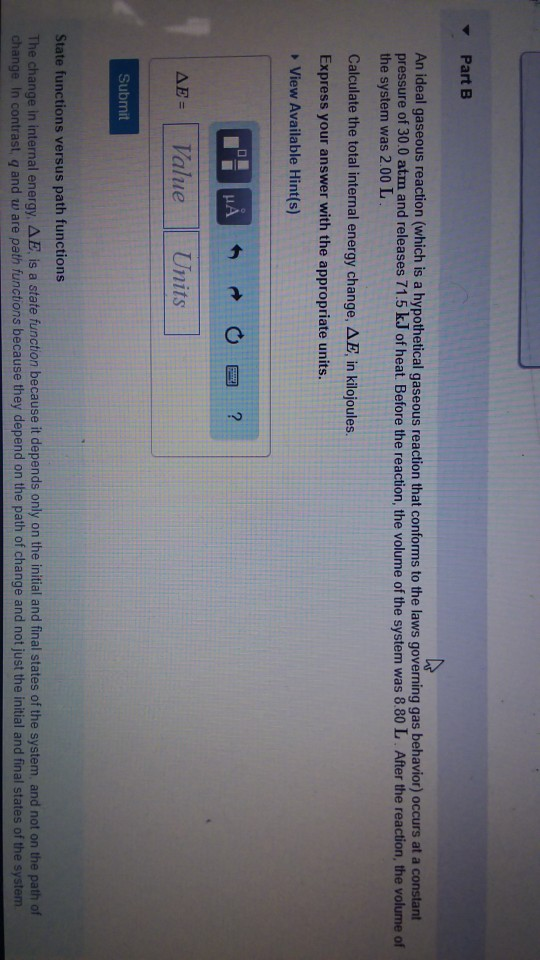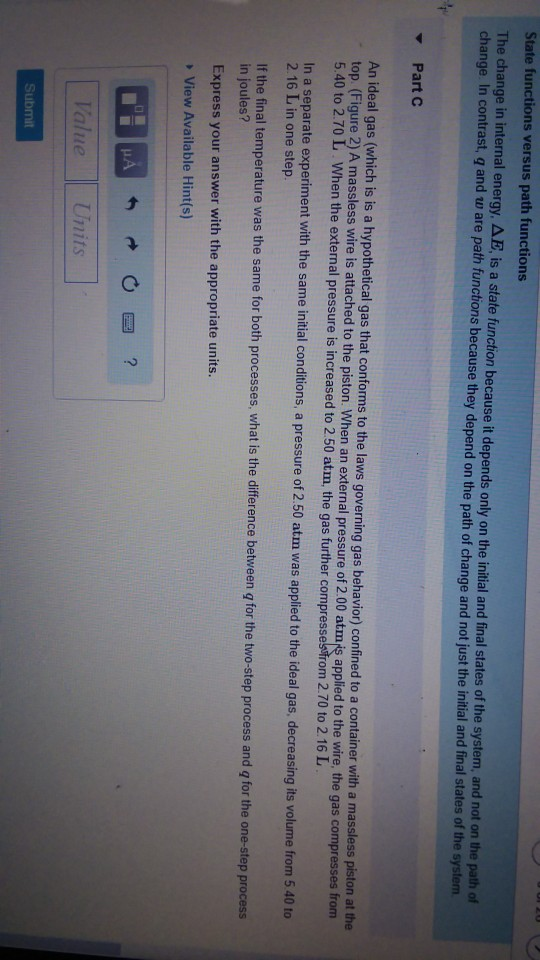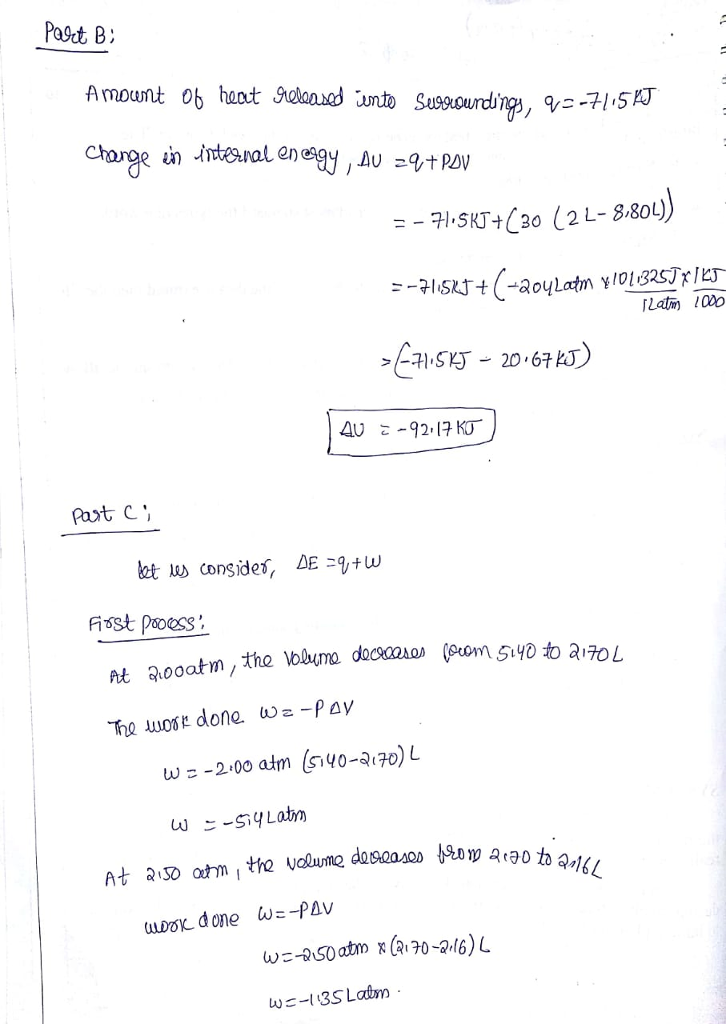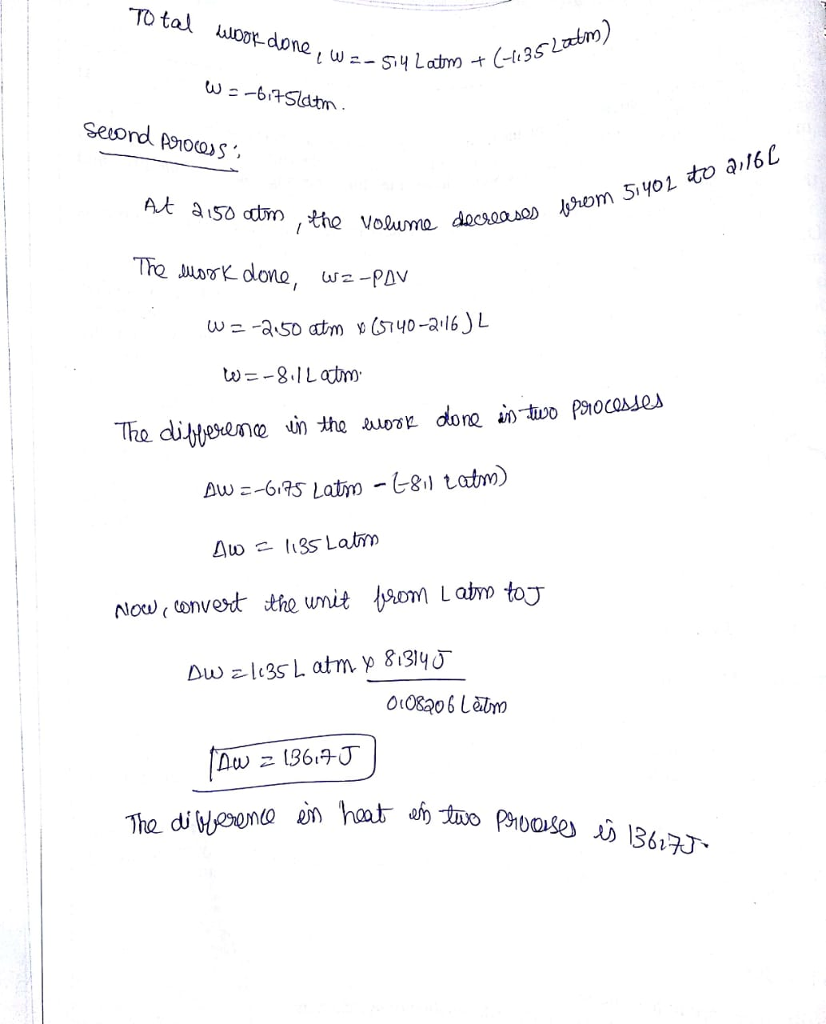#### Earn Coins

Coins can be redeemed for fabulous gifts.

Similar Homework Help Questions
• ### An ideal gaseous reaction (which is a hypothetical gaseous reaction that conforms to the laws governing...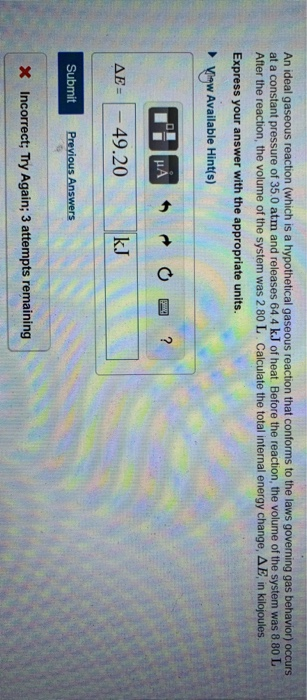An ideal gaseous reaction (which is a hypothetical gaseous reaction that conforms to the laws governing gas behavior) occurs at a constant pressure of 35.0 atm and releases 64.4 kJ of heat. Before the reaction, the volume of the system was 8.80 L After the reaction, the volume of the system was 2.80 L. Calculate the total internal energy change, AE, in kilojoules. Express your answer with the appropriate units. Vigw Available Hint(s) AE = - 49.20 kJ Submit Previous...

• ### Part B An ideal gaseous reaction (which is a hypothetical gaseous reaction that conforms to the...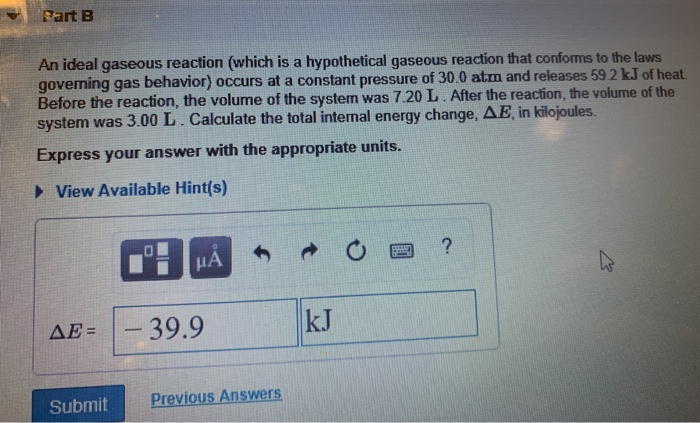Part B An ideal gaseous reaction (which is a hypothetical gaseous reaction that conforms to the laws governing gas behavior) occurs at a constant pressure of 30.0 atm and releases 59.2 kJ of heat. Before the reaction, the volume of the system was 7.20 L. After the reaction, the volume of the system was 3.00 L. Calculate the total internal energy change, AE in kilojoules. Express your answer with the appropriate units. View Available Hint(s) 1: HÅR O a ?...

• ### An ideal gaseous reaction (which is a hypothetical gaseous reaction that conforms to the laws governing...

An ideal gaseous reaction (which is a hypothetical gaseous reaction that conforms to the laws governing gas behavior) occurs at a constant pressure of 50.0 atm and releases 62.9 kJ of heat. Before the reaction, the volume of the system was 6.60 L . After the reaction, the volume of the system was 3.00 L . Calculate the total internal energy change, ΔU, in kilojoules.

• ### An ideal gaseous reaction (which is a hypothetical gaseous reaction that conforms to the laws governing...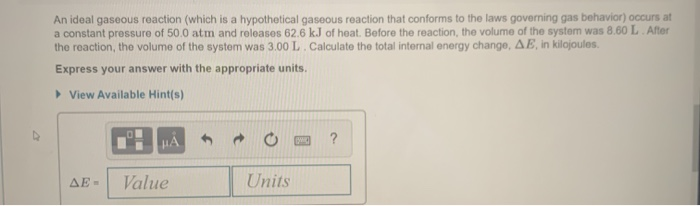An ideal gaseous reaction (which is a hypothetical gaseous reaction that conforms to the laws governing gas behavior) occurs at a constant pressure of 50.0 atm and releases 62.6 kJ of heat. Before the reaction, the volume of the system was 8.60 L After the reaction, the volume of the system was 3.00 L. Calculate the total internal energy change, AE, in kilojoules. Express your answer with the appropriate units. View Available Hint(s) HAO? AE - Value Units

• ### An ideal gaseous reaction (which is a hypothetical gaseous reaction that conforms to the laws governing gas behavior) oc...

An ideal gaseous reaction (which is a hypothetical gaseous reaction that conforms to the laws governing gas behavior) occurs at a constant pressure of 50.0 atm and releases 65.0 kJ of heat. Before the reaction, the volume of the system was 7.60 L . After the reaction, the volume of the system was 2.20 L . Calculate the total internal energy change, ΔE, in kilojoules. Express your answer with the appropriate units.

• ### DIFCIU idue Part B A gaseous reaction occurs at a constant pressure of 35.0 atm and...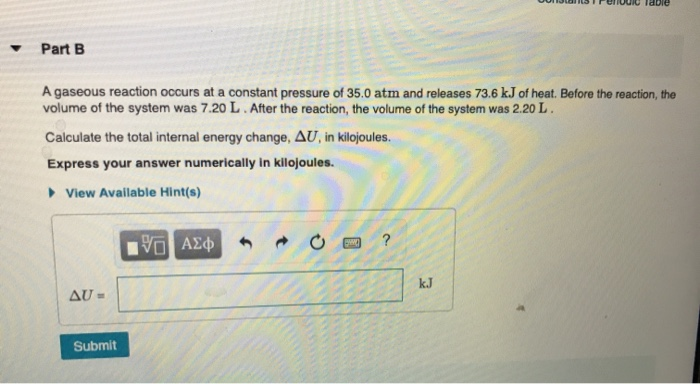DIFCIU idue Part B A gaseous reaction occurs at a constant pressure of 35.0 atm and releases 73.6 kJ of heat. Before the reaction, the volume of the system was 7.20 L. After the reaction, the volume of the system was 2.20 L. Calculate the total internal energy change, AU, in kilojoules. Express your answer numerically in kilojoules. View Available Hint(s) YO AE AU- Submit

• ### 1. Calculate the enthalpy change, ΔH, for the process in which 31.6 g of water is...

1. Calculate the enthalpy change, ΔH, for the process in which 31.6 g of water is converted from liquid at 0.4 ∘C to vapor at 25.0 ∘C . For water, ΔHvap = 44.0 kJ/mol at 25.0 ∘C and Cs = 4.18  J/(g⋅∘C) for H2O(l). 2. How many grams of ice at -16.2 ∘C can be completely converted to liquid at 10.2 ∘C if the available heat for this process is 4.27×103 kJ ? For ice, use a specific heat of 2.01...

• ### Part C An ideal gas (which is is a hypothetical gas that conforms to the laws...

Part C An ideal gas (which is is a hypothetical gas that conforms to the laws governing gas behavior) confined to a container with a massless piston at the top. (Figure 2) A massless wire is attached to the piston. When an external pressure of 2.00 atm is applied to the wire, the gas compresses from 4.90 to 2.45 L . When the external pressure is increased to 2.50 atm, the gas further compresses from 2.45 to 1.96 L ....

• ### An ideal gaseous reaction occurs at a constant pressure of 35.0 atm and releases 55.8 kJ...

An ideal gaseous reaction occurs at a constant pressure of 35.0 atm and releases 55.8 kJ of heat. Before the reaction, the volume of the system was 8.20 L. After the reaction, the volume of the system was 3.01 L. Calculate the total change in internal energy for the system.

• ### An ideal gas (which is is a hypothetical gas that conforms to the laws governing gas...

An ideal gas (which is is a hypothetical gas that conforms to the laws governing gas behavior) confined to a container with a massless piston at the top. (Figure 2) A massless wire is attached to the piston. When an external pressure of 2.00 atm is applied to the wire, the gas compresses from 6.60 to 3.30 L . When the external pressure is increased to 2.50 atm, the gas further compresses from 3.30 to 2.64 L . In a...